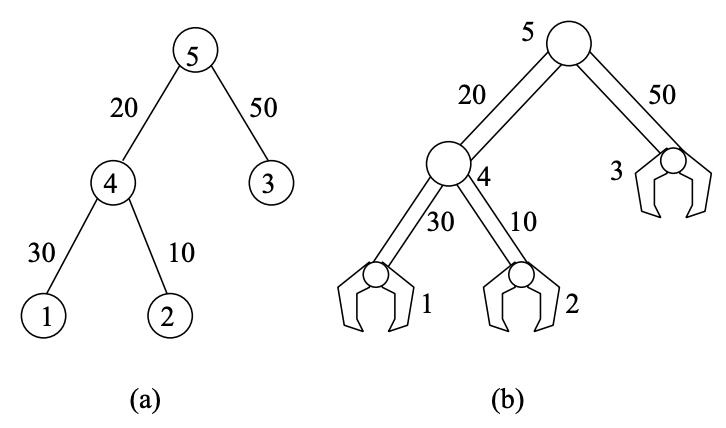시간 제한 메모리 제한 제출 정답 맞은 사람 정답 비율
2 초 512 MB 0 0 0 0.000%

## 문제

Consider a heavy machinery in the form of a binary tree (i.e., every non-leaf node has at most two child nodes) as shown in Figure 1. The edges of the tree are metal bars, which are connected by hinges, denoted by nodes (labelled ‘1’ through ‘5’) in the tree. The leaf nodes of the tree in Figure 1(a) are essentially the claws used by the heavy machinery to perform heavy-duty tasks. The claws are also connected to metal bars by hinges.Figure 1: (a) A tree and (b) the corresponding tree of claws.

As the machinery is load-bearing, the hinges used to connect metal bars must be of the correct grade. For a particular node, say v, in the tree, the grade of the hinge used at that node is determined by the sum of (i) the total weight of the metal bars in the subtree rooted at v and (ii) the total weight of the metal bars from v to the tree root.

The weights of the metal bars are shown beside the corresponding edges in Figure 1. The claws and hinges used in the machinery are made of superlight materials that they can be considered weightless. Hence, the grades of the hinges used at leaf nodes ‘1’, ‘2’, and ‘3’ in Figure 1 to connect the claws to metal bars are 50, 30, and 50, respectively. The grade of the hinge used at node ‘4’ is 60, and the grade of the hinge used at the root node, ‘5’, is 110.

The unladen load of a claw is the sum of the grades of the hinges along the path from the claw to the root node. The unladen loads of the claws in this example are 220 (at ‘1’), 200 (at ‘2’), and 160 (at ‘3’). In this task, you have to output the maximum unladen load among all the claws of a given heavy machinery.

## 입력

Let n (1 ≤ n ≤ 12000) be the total number of nodes in the given binary tree. The input contains n lines. The first line contains the integer n. Each of the next (n − 1) lines contains three integers separated by spaces:

• thisnode, which specifies the node number of the current node.
• parnode, which specifies the node number of the parent of the current node.
• weight, which specifies the weight, a positive integer ≤ 100, of the metal bar connecting the current node and its parent node.

The nodes are labelled 1 through n. The root node is the node without a parent.

## 출력

The output file contains an integer, which is the maximum unladen load among all the claws of a given heavy machinery.

## 예제 입력 1

5
1 4 30
4 5 20
2 4 10
3 5 50


## 예제 출력 1

220